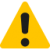# 伤害与抗性

2022-04-25更新

a-c-k-ab-lb## 抗性

### 减少，或"flat"抗性

`M=D-R`

`M=1/(R-D+2)`

`M=1/(R+1)`.

5 0 5
5 1 4
5 2 3
5 3 2
5 4 1
5 5 1/2
5 6 1/3
5 7 1/4
5 8 1/5
... ... ...

### 百分位抗性

`F=M*(1-P)`

```减免抗性/百分比抗性
```

D=30, R=10, 所以 R+1=21<D=30, 然后我们对M使用上面第一个公式：

M=30-10=20

F=20*(1-0.2)=20*0.8=16

## 组合公式

F = (D - R) * (1 - P)

F = (1 - P) / (R-D+2)

F = (1 - P) / (R+1)